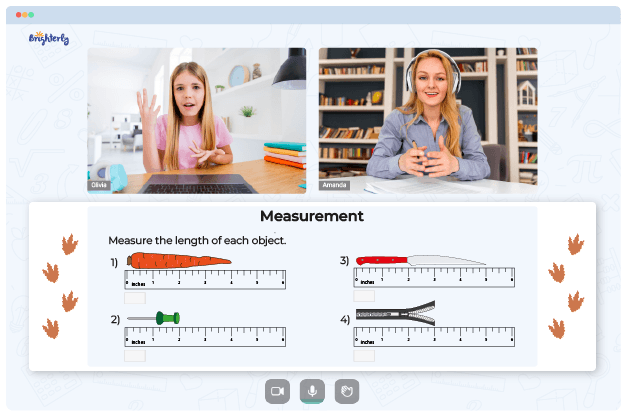# Metric Conversion Worksheets

Every day, kids come across scenarios where they need to measure something to ascertain the object’s weight and length. So, they will always need a generally accepted means of measuring the surrounding objects. And the metric system is used for estimations.

Students can use grams to measure mass, liters for volume, and meters for distance. But sometimes, the concept of metric system conversion can be challenging. Here is how a metric conversion worksheet can help.

## Why You Should Use a Metric Conversion Worksheet from Brighterly

Often, teachers in conventional classrooms are so busy trying to meet the school curriculum that some students may be left behind. Other times, kids might be too shy to ask questions when they do not understand something. Fortunately, with online tutoring, kids now have professionals who can lead them through complicated concepts in an easy and fun way.

Brighterly is an online platform with professional tutors who do their best to give students the best learning experience. When kids have issues understanding metric conversions, the tutors use a unit conversion worksheet to simplify things. First, a metric conversions worksheet introduces kids to the terminologies, like what it means to convert, different metric systems, and lots more.

Math for Kids

Is Your Child Struggling With Math?
1:1 Online Math TutoringThe worksheets’ authors make the resource as easy as possible to use so that kids can have a fun time learning. For instance, a typical converting metric units worksheet can help kids understand that moving a decimal point in the metric system is the most common method of conversion between different units. Also, this worksheet can introduce kids to shortcuts and tricks that allow them to easily convert from kilograms to grams, centimeters to meters, milliliters to liters, etc.

## How to Use a Metric Conversion Worksheet in a Fun Way### Metric Conversion Worksheets PDF

Metric Conversion Worksheet### Metric Conversion Worksheets PDF### Metric Conversion Worksheets PDF

Unit Conversion Worksheet### Metric Conversion Worksheets PDF

Metric Unit Conversion Worksheet

Tutors provide kids with a converting metric units worksheet with answers in PDF format so kids can practice and solidify their understanding of metric conversion. A metric conversion practice worksheet usually has an answer section so kids can always check their progress. Without a metric conversion worksheet answer key, kids might get upset and neglect their studying.

A metric unit conversion worksheet comes in bright colors to attract kids. Also, a unit conversion practice worksheet has activities kids have to complete while studying.

Sometimes, children forget they have a lecture because of numerous activities involved in the studying process. But the advantage is that they subconsciously learn while doing exercises in a unit conversions worksheet.

### Measurement Worksheets

Need help with Measurement?• Does your child need extra support with understanding measurement concepts?
• Start lessons with an online tutor.

Is your child finding it hard to master of measurement? An online tutor could be of help.